# Getting Started

gganimate is an extension of the grammar of graphics, as implemented by the ggplot2 package, that adds support for declaring animations using an API familiar to users of ggplot2.

The following introduction assumes familiarity with ggplot2 to the extend that constructing static plots and reading standard ggplot2 code feels natural. If you are new to both ggplot2 and gganimate you’ll benefit from exploring the trove of ggplot2 documentation, tutorials, and courses available online first (see the ggplot2 webpage for some pointers).

We’ll jump right into our first animation. Don’t worry too much about understanding the code, as we’ll dissect it later.

``````library(gganimate)

p <- ggplot(iris, aes(x = Petal.Width, y = Petal.Length)) +
geom_point()

plot(p)``````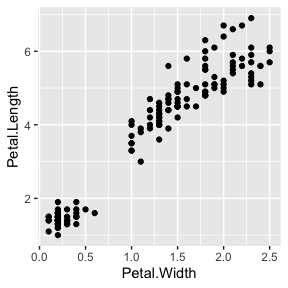You go from a static plot made with ggplot2 to an animated one, simply by adding on functions from gganimate.

``````anim <- p +
transition_states(Species,
transition_length = 2,
state_length = 1)

anim``````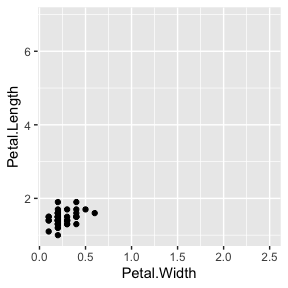`transition_states()` splits up plot data by a discrete variable and animates between the different states.

As can be seen, very few additions to the plot results in a quite complex animation. So what did we do to get this animation? We added a type of transition. Transitions are functions that interpret the plot data in order to somehow distribute it over a number of frames. `transition_states()` specifically splits the data into subsets based on a variable in the data (here `Species`), and calculates intermediary data states that ensures a smooth transition between the states (something referred to as tweening). gganimate provides a range of different transitions, but for the rest of the examples we’ll be sticking to `transition_states()` and see how we can modify the output.

## Easing

When `transition_states()` calculates intermediary data for the tweening, it needs to decide how the change from one value to another should progress. This is a concept called easing. The default easing is linear, but others can be used, potentially only targeting specific aesthetics. Setting easing is done with the `ease_aes()` function. The first argument sets the default easing and subsequent named arguments sets it for specific aesthetics.

``````anim +
ease_aes('cubic-in-out') # Slow start and end for a smoother look``````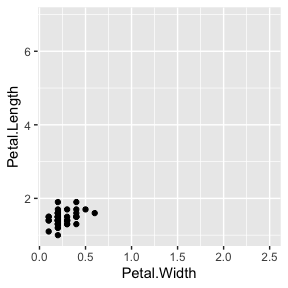`ease_aes()` defines the velocity with which aesthetics change during an animation.

``````anim +
ease_aes(y = 'bounce-out') # Sets special ease for y aesthetic``````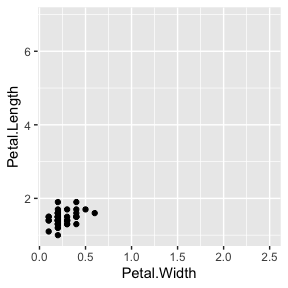## Labeling

It can be quite hard to understand an animation without any indication as to what each time point relates to. gganimate solves this by providing a set of variables for each frame, which can be inserted into plot labels using glue syntax.

``````anim +
ggtitle('Now showing {closest_state}',
subtitle = 'Frame {frame} of {nframes}')``````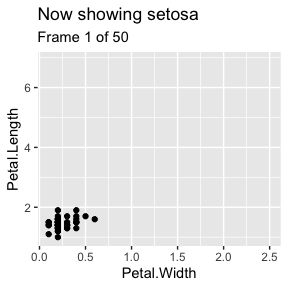❗ Use glue syntax to insert frame variables in plot labels and titles.

Different transitions provide different frame variables. `closest_state` only makes sense for `transition_states()` and is thus only available when that transition is used.

## Object Permanence

In the animation above, it appears as if data in a single measurement changes gradually as the flower being measured on somehow morphs between three different iris species. This is probably not how Fisher conducted the experiment and got those numbers. In general, when you make an animation, graphic elements should only transition between instances of the same underlying phenomenon. This sounds complicated but it is more or less the same principle that governs makes sense to draw a line between two observations. You wouldn’t connect observations from different iris species, but repeated observations on the same plant would be fine to connect. Same thing with animations.

Just to make this very clear (it is an important concept). The line plot equivalent of our animation above is:

``````ggplot(iris, aes(x = Petal.Width, y = Petal.Length)) +
geom_line(aes(group = rep(1:50, 3)), colour = 'grey') +
geom_point()``````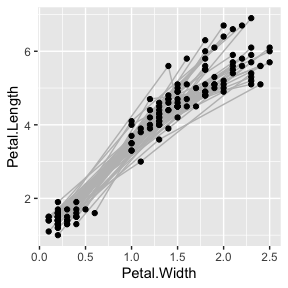Ugh…

So, how do we fix this and tell gganimate to not morph observations from different species into each others? The key is the group aesthetic. You may be familiar with this aesthetic from plotting lines and polygons, but in gganimate it takes a more central place. Data that have the same group aesthetic are interpreted as being linked across states. The semantics of the group aesthetic in ggplot2 is such that if it is undefined it will get calculated based on the interaction of all discrete aesthetics (sans `label`). If none exists, such as in our iris animation, all data will get the same group, and will thus be matched by gganimate. So, there are two ways to fix our plot:

1. Add some aesthetics that distinguish the different species
``````ggplot(iris, aes(x = Petal.Width, y = Petal.Length)) +
geom_point(aes(colour = Species)) +
transition_states(Species,
transition_length = 2,
state_length = 1)``````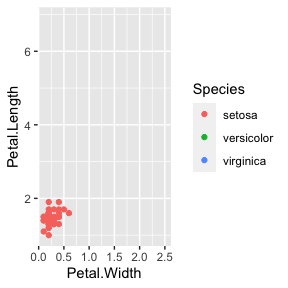1. Set the group directly
``````ggplot(iris, aes(x = Petal.Width, y = Petal.Length)) +
geom_point(aes(group = seq_along(Species))) +
transition_states(Species,
transition_length = 2,
state_length = 1)``````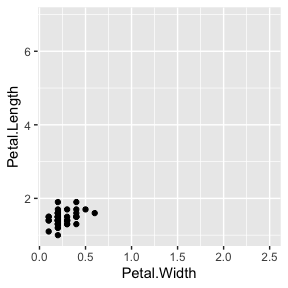❗ The group aesthetic defines how the data in a layer is matched across the animation.

In general 2) is preferred as it makes the intend explicit. It also makes it possible to match data with different discrete aesthetics such as keeping our (now obviously faulty) transition while having different colour for the different species)

``````ggplot(iris, aes(x = Petal.Width, y = Petal.Length)) +
geom_point(aes(colour = Species, group = 1L)) +
transition_states(Species,
transition_length = 2,
state_length = 1)``````